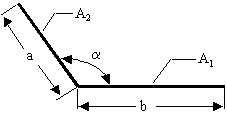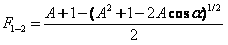Cody

# Problem 2807. Radiation Heat Transfer — View Factors (3)

View factors (aka configuration factors) are utilized in some radiation heat transfer models to estimate heat transfer rates between surfaces. In particular, the thermal energy leaving a given surface is applied to other surfaces, as appropriate, based on how much the hot surface "sees" the other surfaces. As such, view factors are purely geometrical in nature. A range of view factor formulae are available here.

For this problem, calculate the view factor from surface 1 to surface 2 (F_1-2) for two infinitely long plates of different finite widths and having a common edge given the angle between the plates:-Any of the variables can be a vector. Also, note that A = a/b, and is different than A_1 or A_2. Finally, the angle is provided in degrees.

### Solution Stats

41.67% Correct | 58.33% Incorrect
Last Solution submitted on Mar 15, 2020